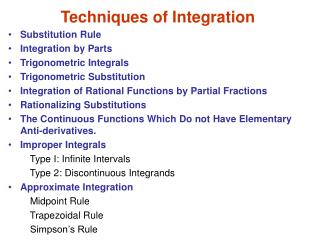DownloadDownload PresentationTechniques of Integration

# Techniques of Integration

Download Presentation## Techniques of Integration

- - - - - - - - - - - - - - - - - - - - - - - - - - - E N D - - - - - - - - - - - - - - - - - - - - - - - - - - -
##### Presentation Transcript

1. Techniques of Integration • Substitution Rule • Integration by Parts • Trigonometric Integrals • Trigonometric Substitution • Integration of Rational Functions by Partial Fractions • Rationalizing Substitutions • The Continuous Functions Which Do not Have Elementary Anti-derivatives. • Improper Integrals Type I: Infinite Intervals Type 2: Discontinuous Integrands • Approximate Integration Midpoint Rule Trapezoidal Rule Simpson’s Rule

2. Strategy for Integration 1. Using Table of Integration Formulas 2. Simplify the Integrand if Possible Sometimes the use of algebraic manipulation or trigonometric identities will simplify the integrand and make the method of integration obvious. 3. Look for an Obvious Substitution Try to find some function in the integrand whose differential also occurs, apart from a constant factor. 3. Classify the Integrand According to Its Form Trigonometric functions, Rational functions, Radicals, Integration by parts. 4. Manipulate the integrand. Algebraic manipulations (perhaps rationalizing the denominator or using trigonometric identities) may be useful in transforming the integral into an easier form. 5. Relate the problem to previous problems When you have built up some experience in integration, you may be able to use a method on a given integral that is similar to a method you have already used on a previous integral. Or you may even be able to express the given integral in terms of a previous one. 6. Use several methods Sometimes two or three methods are required to evaluate an integral. The evaluation could involve several successive substitutions of different types, or it might combine integration by parts with one or more substitutions.

3. Table of Integration Formulas

4. Trigonometric functions

5. Integration by Parts If is a product of a power of x (or a polynomial) and a transcendental function (such as a trigonometric, exponential, or logarithmic function), then we try integration by parts, choosing according to the type of function. Although integration by parts is used most of the time on products of the form described above, it is sometimes effective on single functions. Looking at the following example.

6. Trigonometric Substitution

7. Integration of Rational Functions by Partial Fractions

8. Example Rationalizing Substitutions

9. Approximate Integration

10. The following tables show the results of calculations of but for n=5, 10 and 20 and for the left and right endpoint approximations as well as the Trapezoidal and Midpoint Rules. we see that the errors in the Trapezoidal and Midpoint Rule approximations for are

11. Improper Integrals

12. <